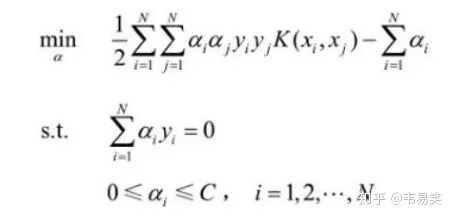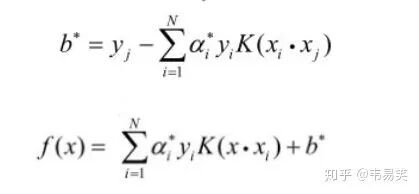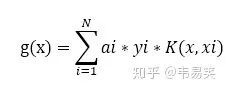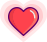### 如何學習SVM？怎麼改進實現SVM演算法程式？答案來了【導讀】在 3D 動作識別領域，需要用到 SVM（支援向量機演算法），但是現在所知道的 SVM 演算法很多很亂，相關的程式包也很多，有什麼方法可以幫助我們更好地理解 SVM 演算法，以及如何利用 matlab 程式設計實現 SVM 演算法，同時在理解原有的程式包以及在原有的程式包基礎上進行改進有什麼好的方法？本文為知乎問題“如何學習 SVM（支援向量機）以及改進實現 SVM 演算法程式？”下的兩個答案。SVM 的問題就變成：求解一系列滿足約束的 alpha 值，使得上面那個函式可以取到最小值。然後記錄下這些非零的 alpha 值和對應樣本中的 x 值和 y 值，就完成學習了，然後預測的時候用：``target = desired output vector``point = training point matrix``procedure takeStep(i1,i2)``   if (i1 == i2) return 0``   alph1 = Lagrange multiplier for i1``   y1 = target[i1]``   E1 = SVM output on point[i1] – y1 (check in error cache)``   s = y1*y2``   Compute L, H via equations (13) and (14)``   if (L == H)``      return 0``   k11 = kernel(point[i1],point[i1])``   k12 = kernel(point[i1],point[i2])``   k22 = kernel(point[i2],point[i2])``   eta = k11+k22-2*k12``   if (eta > 0)``   {``      a2 = alph2 + y2*(E1-E2)/eta``      if (a2 ``      else if (a2 > H) a2 = H``   }``   else``   {``      Lobj = objective function at a2=L``      Hobj = objective function at a2=H``      if (Lobj ``         a2 = L``      else if (Lobj > Hobj+eps)``         a2 = H``      else``         a2 = alph2``   }``   if (|a2-alph2| ``      return 0``   a1 = alph1+s*(alph2-a2)``   Update threshold to reflect change in Lagrange multipliers``   Update weight vector to reflect change in a1 & a2, if SVM is linear``   Update error cache using new Lagrange multipliers``   Store a1 in the alpha array``   Store a2 in the alpha array``   return 1``endprocedure``

``E(i) = f(xi) - yi``

platt 的文章建議是做一個 E 函式的快取，方便後面選擇 i, j 時比較，我看到很多入門版本 svm 實現都是這麼搞得。其實這是有問題的，後面我們會說到。最好的方式是定義一個 g(x) 令其等於：``E(j) = g(xj) + b - yj``

``double Kik = kernel(i, k);``double Kjk = kernel(j, k);``G[k] += delta_alpha_i * Kik * y[i] + delta_alpha_j * Kjk * y[j];``

Platt 的文章裡也證明過一旦某個 alpha 出於邊界（0 或者 C）的時候，就很不容易變動，而且虛擬碼裡也是優先再工作集裡尋找 > 0 and

``K(xi, xj) = xi . xj``

# (*本文為AI科技大本營轉載文章，轉載請聯絡作者)推薦閱讀

• ## 誰說 C++ 的強制型別轉換很難懂？